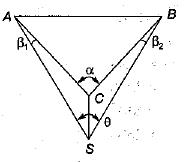Courses

# Tides, Triangulation, Astronomy And Photogrammetric Surveying - 1

## 10 Questions MCQ Test Topicwise Question Bank for GATE Civil Engineering | Tides, Triangulation, Astronomy And Photogrammetric Surveying - 1

Description
This mock test of Tides, Triangulation, Astronomy And Photogrammetric Surveying - 1 for Civil Engineering (CE) helps you for every Civil Engineering (CE) entrance exam. This contains 10 Multiple Choice Questions for Civil Engineering (CE) Tides, Triangulation, Astronomy And Photogrammetric Surveying - 1 (mcq) to study with solutions a complete question bank. The solved questions answers in this Tides, Triangulation, Astronomy And Photogrammetric Surveying - 1 quiz give you a good mix of easy questions and tough questions. Civil Engineering (CE) students definitely take this Tides, Triangulation, Astronomy And Photogrammetric Surveying - 1 exercise for a better result in the exam. You can find other Tides, Triangulation, Astronomy And Photogrammetric Surveying - 1 extra questions, long questions & short questions for Civil Engineering (CE) on EduRev as well by searching above.
QUESTION: 1

Solution:
QUESTION: 2

### For a star at its upper transit, the local sidereal time is equal to

Solution:

When star is at its upper transit, Hour angle (H) is equal to zero.

QUESTION: 3

### The limiting minimum declination of a circumpolar star having latitude 40° N is

Solution:

The declination of circumpolar star is always greater than colatitude of the place of observation, so limiting delineation = colatitude = 90° - 40° = 50°

QUESTION: 4

The relationship between tropical year TY and sidereal year SY is

Solution:

Tropical year = 365.2422 mean solar days.
Sidereal year = 365.2564 mean solar days.

QUESTION: 5

Which one of the following indicates the correct relationship between the flying height (H), the focal length (f), the air base (B) and the photographic base (b)?

Solution:
QUESTION: 6

Satellite station S is established during a triangulation survey as shown in fig.The angle α is equal to

Solution:
QUESTION: 7

Which of the following statements about photogrammetric surveying is correct?

Solution:
QUESTION: 8

Consider the following statements associated with triangulation survey
1. In triangulation survey unknown distances between stations are determined using tacheometric relations.
2. The triangulation station at which astronomical observations are made for azimuths are called Laplace stations
3. In tertiary triangulation, the length of the sides is in the range of 10 to 25 km
4. The length of the base line in primary triangulation is 8 to 12 km Of these statements

Solution:
QUESTION: 9

Given that for a triangulation survey
D = distance in km
h = the visible horizon from a station of known elevation above the datum (in metres) if there is no obstruction due to intervening ground, then h is equal to

Solution:
QUESTION: 10

The sun’s declination remains north in the period between

Solution: